# Stack Class in Java

Learn about Stack Class in Java

Stacks are one of the most useful data structures in programming. Why is stack widely used?

• They are easy to understand
• They reduce the time complexity of certain problems by a huge extent

One of the fundamental techniques used for problem-solving is recursion. Whenever a function is called the memory is allocated to it on a stack. When this function calls itself a new memory is allocated and is placed on the memory of the calling function. This process is executed and understood with the knowledge of stacks.

They find numerous applications in real life problems.

• Mechanism of opening and closing of webpages in a browser
• Wearing/removing of bangles
• Stack of books/plates
• Undo and redo mechanism of text editors
• space for parameters and local variables is created internally using a stack in a program.
• The compiler’s syntax check for matching braces is implemented by using stack.
• Stacks enable us in faster parsing of expressions for evaluation using prefix and postfix expressions

Stacks follow a certain order for adding and removing data (LIFO). In this article we will discuss various aspects of stack and how do we implement them using java language

## What is Stack in Java?

A stack is a linear data structure. It provides a container to store data items which can be accessed from one side of the container only. Here the element that is inserted at last is deleted first.

This is called the Last-In-First-Out (LIFO) principle.

It means that we can only access the latest entered elements.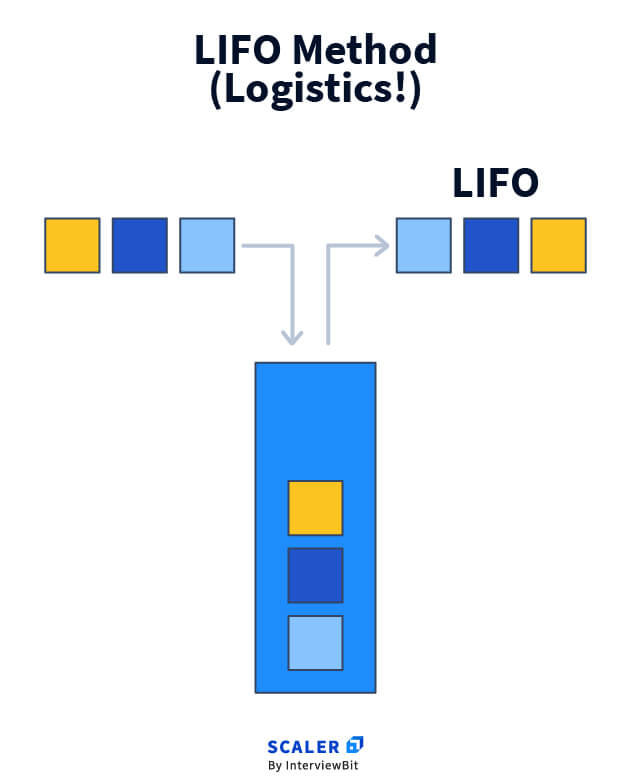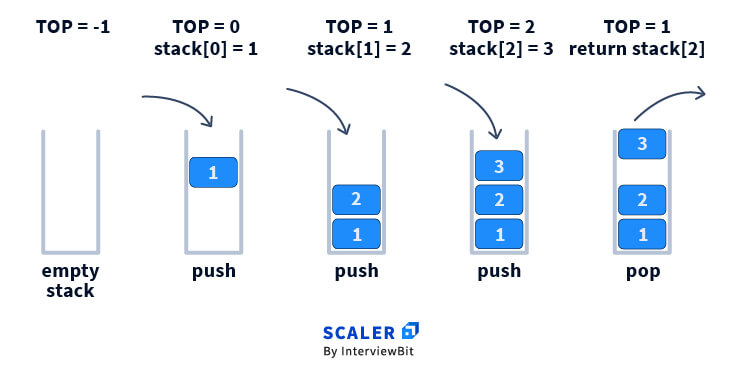## Java Stack Implementation

The concept of stacks can be implemented in multiple ways.

In Java, we can implement stacks in the following ways

### 1. Stacks can be implemented using arrays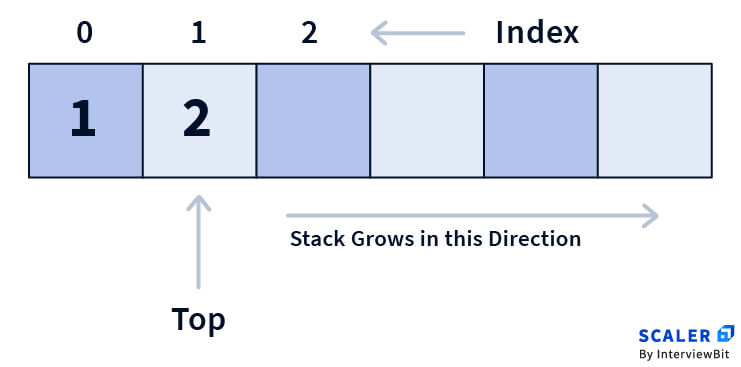• We declare an empty array of specific size in this process.
• We declare a variable top to constantly point to the top element of the stack. Initially it is equal to -1 and denotes underflow (stack is empty and hence we cannot delete anything).
• When we want to add an element to the stack, we first increment the top and then add the element at the index ‘top’.
• When we want to remove the top element we decrease top by 1.
• When the ‘top’ equals array size it declares overflow(stack is filled and we cannot add a new value).

### 2. Stacks can be implemented using linked list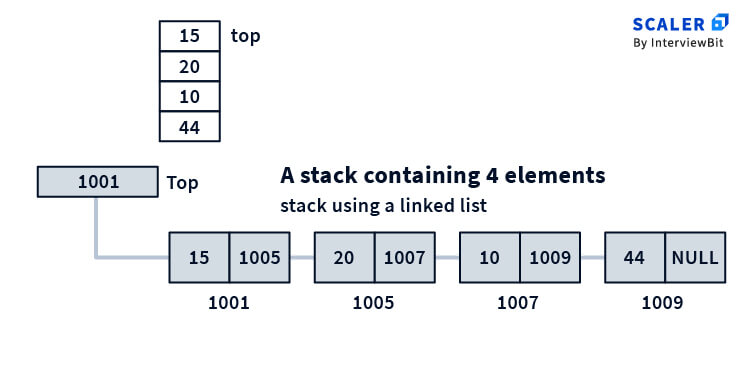• We create a node pointer named head to point to the top of the stack.
• We initialise head with null to denote empty stack.
• When we want to add an element we update the head with the new element’s node address.We make the new node’s next pointer point to the previous address stored in head.
• When we want to remove the top element we just update the head pointer with the address of the top element points to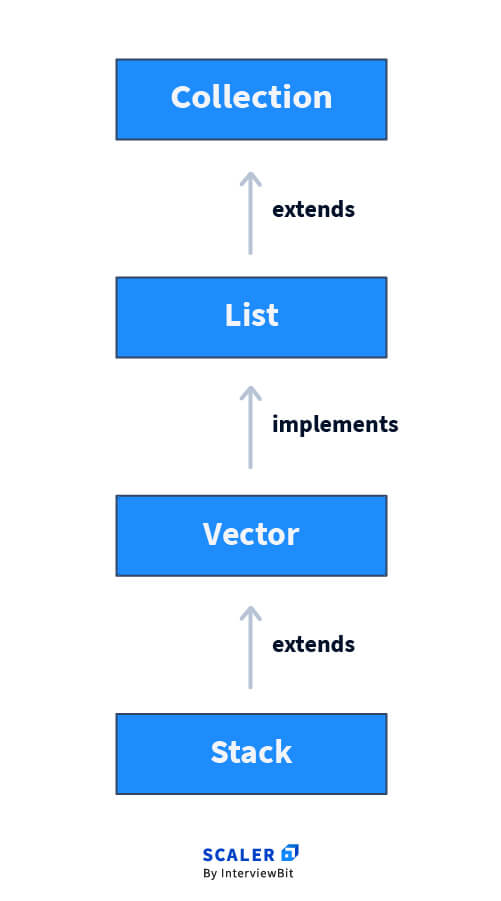Stacks can be implemented using Stack class provided to us by Java package

• Here we import the stack class in a java package named ‘util’ and then implement it with data types like integer, string, char etc.
• We can also implement stacks here for user defined data types.

## Creating a Java stack

For implementing stacks using the Stack class provided by the java packages we need to first import a ‘util’ package.

### Syntax

``````import java.util.Stack (this specifically provides us stack class)
import java.util.* (this provides many classes to us including stack class)

``````

After importing the stack class we need the syntax to declare the stack variable and dynamically allocate memory for it.

### Syntax

``````Stack<data type> stack_name = new Stack<>();

``````
``````// importing stack class from util package
import java.util.Stack;
public class stackDemo
{
public static void main(String[] args) {
//declaration of stack
Stack<Integer> s = new Stack<>();

//insert elements into stack
s.push(10);
s.push(20);
s.push(30);
s.push(40);
s.push(50);

//printing and deleting top element of stack
while(!s.empty()) {
System.out.println(s.peek());
s.pop();
}
}
}

``````

### Output:

``````PS C:\MinGW\bin> Java stackDemo.java
PS C:\MinGW\bin> Java stackDemo
50
40
30
20
10
PS C:\MinGW\bin>[]

``````

## Java Stack Methods

### 1) push()

This method inserts a given value into the stack at the top

``````Stack<Integer> s = new Stack<>();

//insert elements into stack
s.push(10);
s.push(20);
s.push(30);

//printing and deleting top element of stack
while(!s.empty()) {
System.out.println(s.peek());
s.pop();
}

``````

### Output:

``````PS C:\MinGW\bin> Java stackDemo.java
PS C:\MinGW\bin> Java stackDemo
30
20
10
PS C:\MinGW\bin>[]

``````

### 2) pop()

This method removes the top element from the stack and returns it until the stack becomes completely empty

``````// importing stack class from util package
import java.util.Stack;
public class stackDemo
{
public static void main(String[] args) {
//declaration of stack
Stack<Integer> s = new Stack<>();

//insert elements into stack
s.push(10);
s.push(20);
s.push(30);

//pop function returns and deletes thhe top element
System.out.println(s.pop());
}
}

``````

### Output:

``````PS C:\MinGW\bin> Java stackDemo.java
PS C:\MinGW\bin> Java stackDemo
30
PS C:\MinGW\bin>[]

``````

### 3) peek()

This method returns us the top element of the stack. It throws an error when we use it with an empty stack and tells us underflow.

(Underflow : Condition where our stack becomes empty)

``````// importing stack class from util package
import java.util.Stack;
public class stackDemo
{
public static void main(String[] args) {
//declaration of stack
Stack<Integer> s = new Stack<>();

//insert elements into stack
s.push(10);
s.push(20);
s.push(30);

//returning top element
System.out.println(s.peek());

while(!s.empty())s.pop();
System.out.println(s.peek());
}
}

``````

### Output:

``````PS C:\MinGW\bin> Java stackDemo.java
PS C:\MinGW\bin> Java stackDemo
30
at java.base/java.util.Stack.peek(Stack.java:101)
at StackDemo.main(stackDemo.java:18)
PS C:\MinGW\bin>[]

``````

### 4) search()

This method is used to know whether an element exists in our stack or not.

If the element exists it returns the position of that element from the top of the stack.

If the element is absent it returns -1.

``````// importing stack class from util package
import java.util.Stack;
public class stackDemo
{
public static void main(String[] args) {
//declaration of stack
Stack<Integer> s = new Stack<>();

//insert elements into stack
s.push(10);
s.push(20);
s.push(30);

//searching if an element exists
System.out.println(s.search(20));
System.out.println(s.search(50));
}
}

``````

### Output:

``````PS C:\MinGW\bin> Java stackDemo.java
PS C:\MinGW\bin> Java stackDemo
2
-1
PS C:\MinGW\bin>[]

``````

### 5) empty()

This method returns a boolean value.

If the stack is empty it returns true otherwise it returns false.

``````// importing stack class from util package
import java.util.Stack;
public class stackDemo
{
public static void main(String[] args) {
//declaration of stack
Stack<Integer> s = new Stack<>();

//checks if stack is empty
System.out.println(s.empty());

//insert elements into stack
s.push(10);
s.push(20);
s.push(30);

//checks if stack is empty
System.out.println(s.empty());
}
}

``````

### Output:

``````stackDemo.java
PS C:\MinGW\bin> Java stackDemo
true
false
PS C:\MinGW\bin>[]

``````

## Conclusion

Hence it can be concluded that stacks are one of the most relevant data structures to study. It’s application in various real life domains helps us to correlate easily with any new problem.

Their implementation saves us a lot of time in many problems. For example:

Next Greater Element Problem

Here we are expected to find the first greater element to the right of every element present in the array:

``````A=[1,4,3,7,9,5,2,8];
Solution =[4,7,7,9, ,8,8 ];

``````

Now the brute force approach here is to iterate the array for every index and find the first greater element. This approach gives us complexity of O(n2).

Whereas the same is possible to do with the use of a stack in complexity of O(n)m, which is a huge improvement from the brute force approach.

Stacks are also implemented in allocating memory in programs. Its implementation enables us to study and practice recursion.